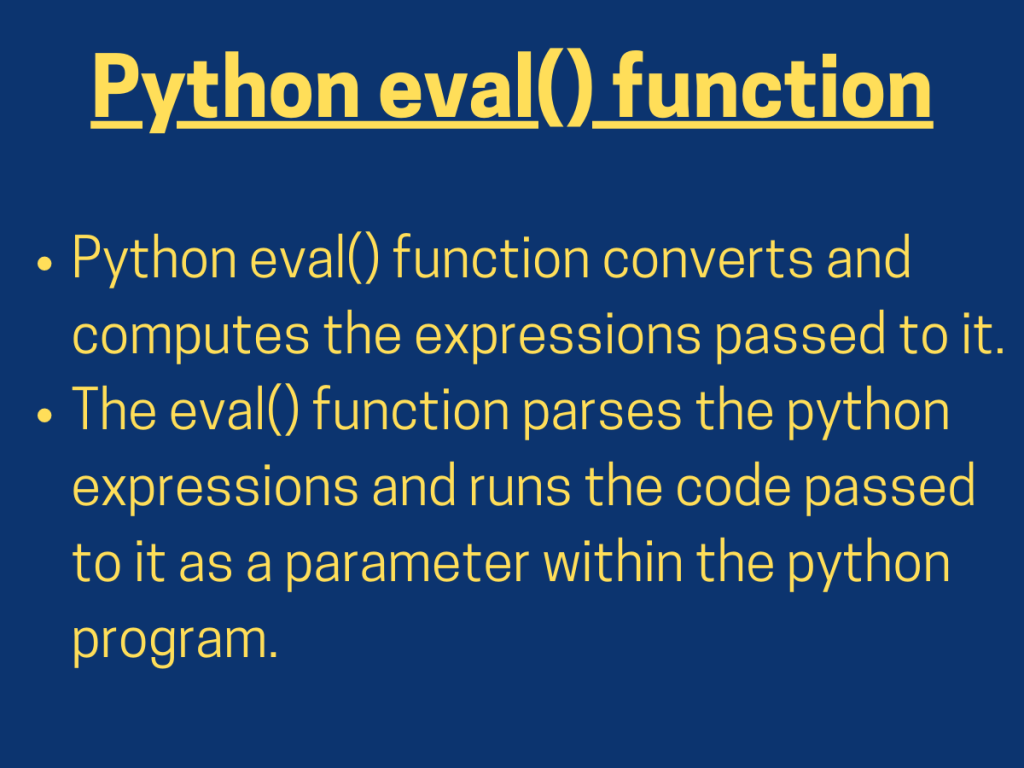# Python eval() function## Understanding Python eval() function

Python eval() function converts and computes the expressions passed to it.

NOTE: Use this method only for testing purposes. The eval() function does not sanitize the expressions passed to it. It can easily become a loophole into your server if malicious users execute Python code here.

The `eval() function` parses the python expressions and runs the code passed to it as a parameter within the python program.

Syntax:

```eval('expression', globals=None, locals=None)
```
• `expression`: It can be any python expression(string parameter) that the user wants to compute within the python code itself.
• `globals`: It is a dictionary that specifies the expressions available for the eval() function to execute.
• `locals`: It describes the local methods and data variables that the eval() function can avail.

Example 1: Passing expression to add two local variables

```a=20
b=30
res = eval('a+b')
print(res)
```

In the above snippet of code, we have passed an expression ‘a+b’ to the eval() function in order to add two local variables: a, b.

Output:

```50
```

Example 2: Python eval() function with user input

```num1 = int(input())
num2 = int(input())
mult = eval('num1*num2')
print('Multiplication:',mult)
```

In the above example, we have accepted the input from the user and assigned the same to the variables. Further, we have passed the expression for the multiplication of those two input values.

Output:

```30
20
Multiplication: 600
```

## Python eval() function with Pandas module

Python eval function can also operate with Pandas Module. The pandas.eval() function accepts the expression and executes the same within the python program.

Syntax:

```DataFrame.eval('expression',inplace)
```
• `expression`: The python expression enclosed within the string quotes to be executed within the python program.
• `inplace`: Default value=TRUE. If the python expression turns out to be an assignment expression, inplace decides to perform the operation and mutate the data frame object. If FALSE, a new dataframe gets created and is returned as a result.

Example 1: Passing an expression with inplace = TRUE

```import pandas
data = pandas.DataFrame({"x":[1,2,3,4],"y":[1,3,5,7],"w":[2,4,6,8],"z":[1,1,1,1]})
print("Original data:\n")
print(data)
data.eval('z = x * y', inplace = True)
print("Data after executing eval():\n")
print(data)
```

In the above example, we have created a dataframe and have passed an expression to be executed within the python script.

As the inplace is set to TRUE, the data values obtained from the expression will be stored in the same dataframe object ‘data’.

Output:

``` Original data:

x  y  w  z
0  1  1  2  1
1  2  3  4  1
2  3  5  6  1
3  4  7  8  1

Data after executing eval():

x  y  w   z
0  1  1  2   1
1  2  3  4   6
2  3  5  6  15
3  4  7  8  28
```

Example 2: Executing expression within python script with inplace = FALSE

```import pandas
data = pandas.DataFrame({"x":[1,2,3,4],"y":[1,3,5,7],"w":[2,4,6,8],"z":[1,1,1,1]})
print("Original data:\n")
print(data)
data1 = data.eval('z = x * y', inplace = False)
print("Data after executing eval():\n")
print(data1)
```

In the above snippet of code, we have passed inplace = FALSE to the eval() function. Thus, the results of the python expression will be stored in a new dataframe object ‘data1’.

Output:

```Original data:

x  y  w  z
0  1  1  2  1
1  2  3  4  1
2  3  5  6  1
3  4  7  8  1
Data after executing eval():

x  y  w   z
0  1  1  2   1
1  2  3  4   6
2  3  5  6  15
3  4  7  8  28
```

## Security issues with eval() function

• Python eval() function is more prone to security threats.
• The sensitive user input data can easily be made available through Python eval() function.
• Thus, the parameters globals and locals is provided to restrict the access to the data directly.

## Summary

• Python eval() function is used to execute python expressions within a python script directly.
• The eval() function can also be used in a similar fashion with the Pandas module.
• Python eval() function is more prone to security threats. Thus, it is necessary to check the information being passed to the eval() function before it’s execution.

## Conclusion

Therefore, in this article, we have understood the working and the vulnerabilities of Python eval() function.

## References

• Python eval() function — JournalDev